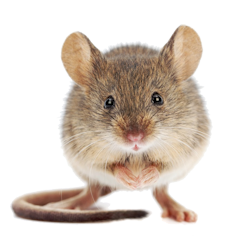Games
Problems
Go Pro!

# Cat and Mouse: Direct and inverse proportion

Lesson Plans > Mathematics > Algebra > Variation

## Cat and Mouse: Direct and inverse proportionThe 'Cat and Mouse' problem typically appears as a brainteaser or puzzle, rather than as a math problem. The reason for this is that the 'obvious' answers are not correct, so the problem (although simple from a mathematical standpoint) seems to be a perplexing brainteaser.

The Cat and Mouse problem is generally stated in the following manner (although the numbers may be different in different versions of the problem):

If 5 cats can catch 5 mice in 5 days, how many days does it take 3 cats to catch 3 mice?

The obvious answer is three. Three cats catch three mice in three days. However, this answer is not correct.

The problem is actually a great opportunity to teach direct variation and inverse variation.
Direct variation means that as one quantity increases, the other quantity will also increase. For example: as the number of cats increases the number of mice they can catch increases proportionately.

Inverse variation is just the opposite. As one quantity increases, the other quantity decreases. For example: as the number of cats increases the number of hours required to catch a certain number of mice decreases.

With these two items under our belts, we can attack the cat and mouse problem in a manner that not only gives us the correct solution, but also gives our students the opportunity to practice these two concepts of direct proportion and inverse proportion.

First, we ask "If 5 cats can catch 5 mice in 5 days, how many mice can they catch in one day?"

Well, they have one fifth as much time, so it stands to reason they will catch one fifth as many mice. 5 cats catch 1 mouse in 1 day.

Now, if 5 cats catch 1 mouse in 1 day, How many days would it take 1 cat to catch 1 mouse?

This is an inverse proportion. If you have less cats, it takes more time to catch the mice. In fact, for one-fifth as many cats, it takes five times as many days to do the task. 1 cat catches 1 mouse in 5 days.

And finally, since 1 cat catches 1 mouse in 5 days, if you have three times as cats, they'll catch three times as many mice in the same amount of time. So 3 cats catch 3 mice in 5 days, which is the answer to the problem.

The 'trick' (if you can call it a trick) to the solution was to always hold one of the three quantities (cats, mice, days) constant, while varying the other two.

Here's a slightly more complicated variation on this problem, with solution acheived in a similar manner:

If a boy and a half can mow a lawn and a half in a day and a half, how many days will it take 5 boys to mow 20 lawns?

Again, the number of lawns mowed is directly proportional to the number of boys, the number of days is inversely proportional to the number of boys, etc.

Let's start out by getting rid of some decimals, and again notice that in each step we keep one quantity constant while varying the other two. To make this obvious, the constant quantity is printed in bold.

1.5 boys mow 1.5 lawns in 1.5 days, so...

3 boys mow 3 lawns in 1.5 days
3 boys mow 6 lawns in 3 days
1 boy mows 2 lawns in 3 days
5 boys mow 10 lawns in 3 days
5 boys mow 20 lawns in 6 days, which is the answer.

We have solved both the cat-and-mouse problem and the boys-and-lawns problems without writing a single algebraic expression. The value of this is, the students are forced to understand why it works, rather than just trying to plug in values into an equation they don't really understand. Then, once they are comfortable with this manipulation, they will be ready for equations such as:

d =
kl
b
, or:

days = k *
number of lawns
number of boys

Lesson by Mr. TwitchellLike us on Facebook to get updates about new resources